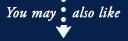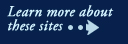Math Activities - Apple Education - Apples and More - University of Illinois Extension# Apples: A Class Act

## Math Activities

1. Apple Fractions

Arrange for students to work in pairs. Give each pair a piece of drawing paper, an apple and a knife. Ask students to think of how many different ways they can slice or cut up an apple so that all pieces are of equal size and shape. Students first draw several different ways and then choose one way and actually cut their apples.

Discuss with the class the variety of ways that apples can be divided into equal parts. On the chalkboard list the fractions that show the different ways that children divided their apples.

Students can also write apple problems using the fractions that were listed on the board after the activity. For example: Sara divided an apple into eighths. She gave 3/8 to Mary. How much of her apple did she have left?

2. Symmetry

Direct students to cut an apple in half and compare the halves. Are the halves symmetrical? Ask children to draw the inside halves of their apples, taking care to include details.

3. Estimating

Have children estimate how many apples would be needed to fill in an outline of their bodies. Ask for a volunteer to be traced on bulletin board paper. Have other volunteers cut out apples of approximate actual size from construction paper. After students make their estimate, see how many "fill in" the shape of the body.

4. Writing Math Problems

Students can write word problems using apples as the topic. The problems can involve many different kinds of computation, depending on what the class is currently studying, for example: multiplication, division, addition, subtraction, fractions, percentages, and ratio.

5. Graphics

Ask students to bring their favorite kind of apple. (Tell them to make sure they know what variety they have.) Make a bar graph listing each apple variety that was represented in the activity and how many times each variety was chosen as the favorite.

6. Circumference

Using string, find the circumference of an apple.